SCHOLASTIC APTITUDE TEST 1999

MATHEMATICS

 Time: Two Hours Max. Marks: 60

• Answers must be written in English or the medium of instruction of the candidate in High school.
• Attempt all questions.
• Answer all the questions in the booklets provided for the purpose. No pages should be removed from the booklets.
• There is no negative marking.
• Answer all questions of section I at one place. Same applies to section II. The remaining questions can be answered in any order.
• Answers to sections I and II must be supported by mathematical reasoning.
• Use of calculators, slide rule, graph paper and logarithmic, trigonometric and statistical tables is not permitted

Section I

This section has 5 questions. Each question is provided with five alternative answers. Only one of them is correct. Indicate the correct answer by A or B or C or D or E. Order of the questions must be maintained. (5x2=10 Marks)

1. Let R be the set of all real numbers. The number of functions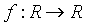satisfying the relation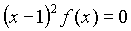is
 A) Infinite B) One C) Two D) Zero E) None of the above
1. a is a number such that the exterior angle of a regular polygon measures 10a degrees. Then
 A) there is no such a B) there are infinitely many such a B) there are precisely nine such a D) there are precisely seven such a E) there are precisely ten such a
1. f(n)=2f(n–1)+1 for all positive integers n. Then
 A)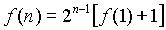B)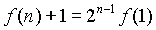C)D)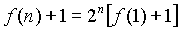E) None of these
1. For any triangle let S and I denote the circumcentre and the incentre respectively. Then SI is perpendicular to a side of
 A) any triangle B) no triangle C) a right angled triangle D) an isosceles triangle E) an obtuse angled triangle
1. If f(x)=x3+ax+b is divisible by (x-1)2 , then the remainder obtained when f(x) is divided by x+2 is
 A) 1 B) 0 C) 3 D) –1 E) None of these

Section II

This section has 5 questions. Each question is in the form of a statement with a blank. Fill the blank so that the statement is true. Maintain the order of the questions. (5x2=10 Marks)

1.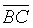is a given line segment, H, K are points on it such that BH=HK=KC. P is a variable point such that
(i)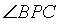has the constant measure of
(ii)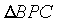has counter clockwise orientation
Then the locus of the centroid ofis the arc of the circle bounded by the chord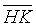with angle in the segment ____________ radians.
2. The coefficient of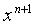in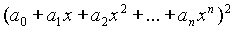is __________
3. n is a natural number such that
i) the sum of its digits is divisible by 11
ii) its units place is non-zero
iii) its tens place is not a 9.
Then the smallest positive integer p such that 11 divides the sum of digits of (n+p) is _____________
4. The number of positive integers less than one million (106) in which the digits 5, 6, 7, 8, 9, 0 do not appear is ______________
5. The roots of the polynomial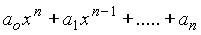are all positive and are denoted by a i, for i=1, 2, 3, ….., n. Then the roots of the polynomial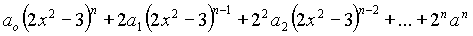are, in terms of
a i, _________.

Section III

This section has 5 questions. The solutions are short and methods, easily suggested. Very long and tedious solutions may not get full marks. (5x2=10 Marks)

1. Given any integer p, prove that integers m and n can be found such that p=3m+5n.
2. E is the midpoint of side BC of a rectangle ABCD and F the midpoint of CD. The area of D AEF is 3 square units. Find the area of the rectangle.
3. If a, b, c are all positive and c¹ 1, then prove that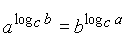4. Find the remainder obtained when x1999 is divided by x2–1.
5. Remove the modulus :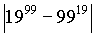Section IV

This section has 6 questions. The solutions involve either slightly longer computations or subtler approaches. Even incomplete solutions may get partial marks. (6x5=30 Marks)

1. Solve the following system of 1999 equations in 1999 unknowns :
x1+x2+x3=0, x2+x3+x4=0……., x1997+x1998+x1999=0,
x
1998+x1999+x1=0, x1999+x1+x2=0
2. Given base angles and the perimeter of a triangle, explain the method of construction of the triangle and justify the method by a proof. Use only rough sketches in your work.
3. If x and y are positive numbers connected by the relation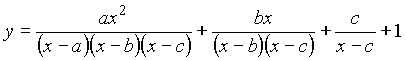, prove that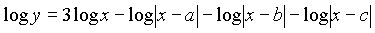for any valid base of the logarithms.
4. Let D XYZ denote the area of triangle XYZ. ABC is a triangle. E, F are points on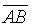and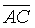respectively.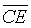and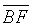intersect in O. If D EOB=4, D COF=8, D BOC=13, develop a method to estimate D ABC. (you may leave the solution at a stage where the rest is mechanical computation).
5. Prove that 80 divides6. ABCD is a convex quadrilateral. Circles with AB, BC, CD, DA as a diameters are drawn. Prove that the quadrilateral is completely covered by the circles. That is, prove that there is no point inside the quadrilateral which is outside every circle.